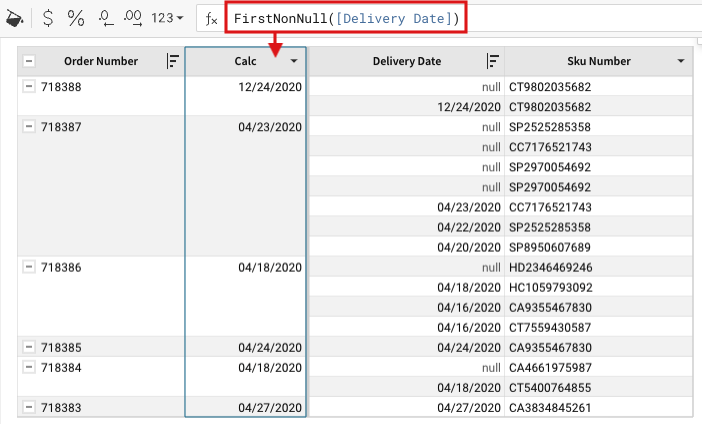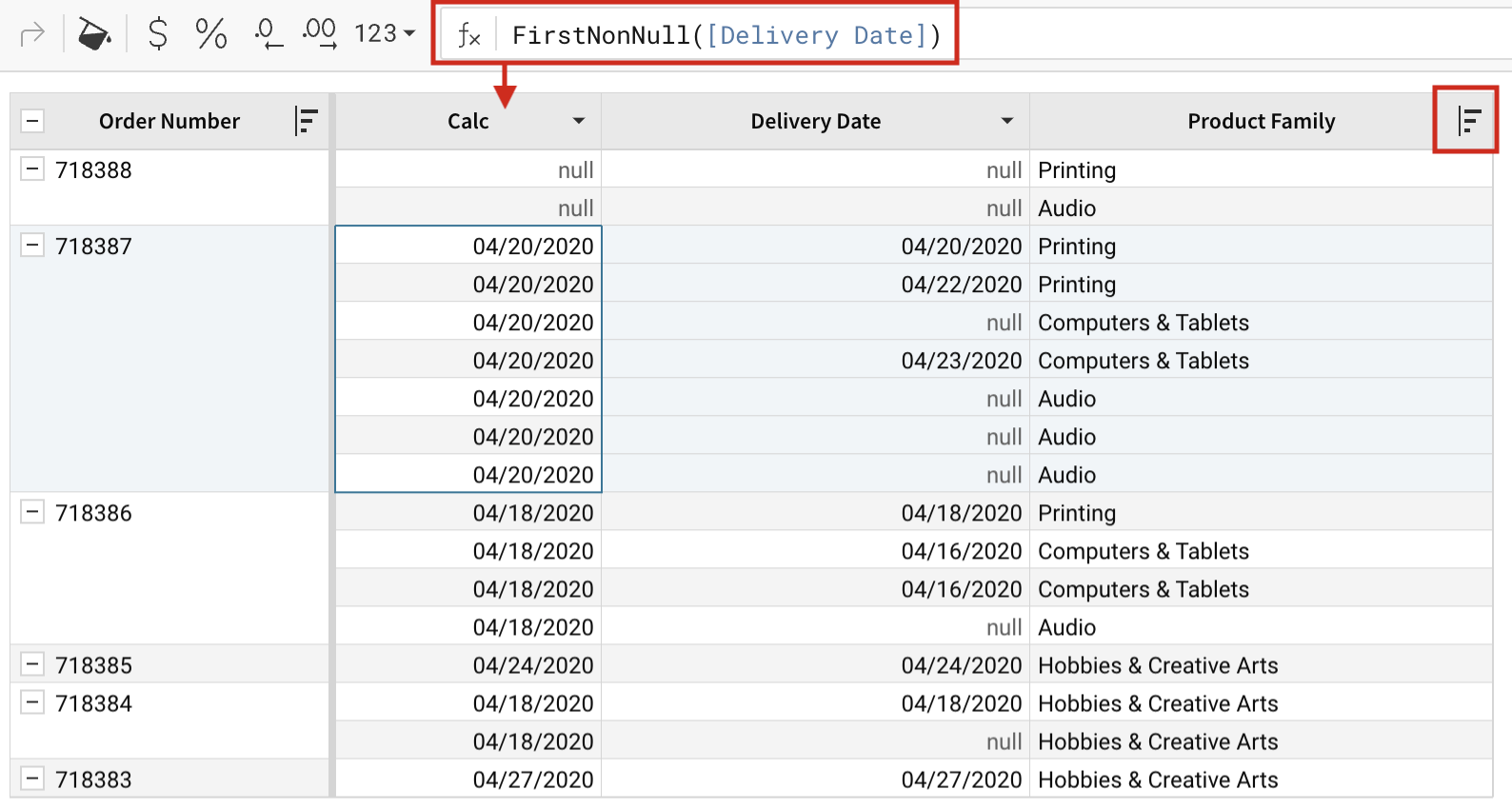# FirstNonNull

Returns the first non-null value in a column or grouping.

FirstNonNull() can be used as either an aggregate function or a window function. When used as a window function, it repeats the first non-null value for every row in the column.

This is similar to the First() function but excludes null values.

## Syntax

`FirstNonNull(value)`

## Arguments

 Term Description value A column to reference or a formula to calculate. The first non-null value is derived from input.  All column types are accepted.

## Notes

It's important to consider data sort order when using this function:

• All sorting and/or filtering is applied prior to calculating this function.
• If no sort order is explicitly set, Sigma doesn't force one. In this case, sort order is determined by your Cloud Data Warehouse.

## Examples

### Example #1

The example below shows the most recent non-null date from the [Delivery Date] column for each grouping under the [Order Number] column.

`FirstNonNull([Delivery Date])`Note how [Delivery Date] is sorted descending. If, instead [Delivery Date], was sorted ascending, the function would return the least recent non-null date for each grouping.

### Example #2

The example below is much like Example #1. However, instead of using FirstNonNull() as an aggregate function, we use it as a window function. This means the calculation references a column in the same group, instead of referencing a column in a lower group. As a result the first non-null value is returned for every row.

Also, note how the resulting calculations are dependent on the group’s sort order. In this example, the group of columns in which FirstNonNull() is calculated is sorted by the [Product Family] column. This sort order impacts the order of the [Delivery Date] column, which is then passed to FirstNonNull() in the [Calc] column.